Most Affordable JEE | NEET | 8,9,10 Preparation by Kota's Top IITian Doctor Faculties

# NCERT Solutions for Class 12 Physics Chapter 10 Wave Optics PDF - eSaral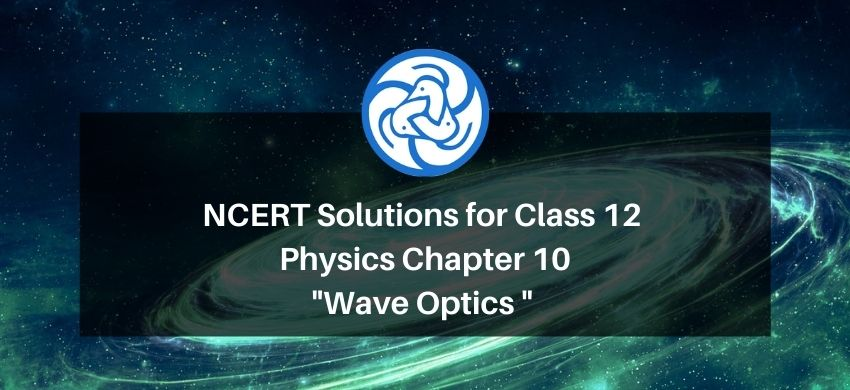# NCERT Solutions for Class 12 Physics Chapter 10 Wave Optics PDF

Hey, are you a class 12 student and looking for ways to download NCERT Solutions for Class 12 Physics Chapter 10 Wave Optics PDF? If yes. Then read this post till the end.

In this article, we have listed NCERT Solutions for Class 12 Physics Chapter 10 Wave Optics in PDF that are prepared by Kota’s top IITian Faculties by keeping Simplicity in mind.

If you want to learn and understand class 12 Physics Chapter 10 "Wave Optics" in an easy way then you can use these solutions PDF.

NCERT Solutions helps students to Practice important concepts of subjects easily. Class 12 Physics solutions provide detailed explanations of all the NCERT questions that students can use to clear their doubts instantly.

If you want to score high in your class 12 Physics Exam then it is very important for you to have a good knowledge of all the important topics, so to learn and practice those topics you can use eSaral NCERT Solutions.

So, without wasting more time Let’s start.

### Download NCERT Solutions for Class 12 Physics Chapter 10 Wave Optics PDF

Question 1. Monochromatic light of wavelength $589 \mathrm{~nm}$ is incident from air on a water surface. What are the wavelength, frequency and speed of

(a) reflected, and

(b) refracted light? Refractive index of water is $1.33$

Solution. The wavelength of incident monochromatic light,

$\lambda=589 \mathrm{~nm}=589 \times 10^{-9} \mathrm{~m}$

Speed of light in air, $c=3 \times 10^{8} \mathrm{~m} / \mathrm{s}$

Refractive Index of water, $\mu=1.33$

(a) The reflected ray will reflect back in the same medium as that of the incident ray medium. Hence, the

wavelength, frequency, and speed of the reflected ray will be the same as that of the Incident ray because of the same medium.

Frequency of light is,

$v=\frac{c}{\lambda}$

$=\frac{3 \times 10^{8}}{589 \times 10^{-9}}$

$=5.09 \times 10^{14} \mathrm{~Hz}$

Hence, wavelength, frequency, and speed of the reflected light in the same medium are $589 \mathrm{~nm}, 5.09 \times 10^{14} \mathrm{~Hz}$, and $3 \times 10^{8} \mathrm{~m} / \mathrm{s}$ respectively.

(b) Frequency of light does not depend on the travelling medium. Therefore, the frequency of the refracted ray in water is equal to the frequency of the incident or reflected light in air.

Refracted frequency, $\mathrm{v}=5.09 \times 10^{14} \mathrm{~Hz}$

Speed of light in water is:

$v=\frac{c}{\mu}$

$\mathrm{v}=\frac{3 \times 10^{8}}{1.33}=2.26 \times 10^{8} \mathrm{~m} / \mathrm{s}$

wavelength of light in water is,

$\lambda=\frac{v}{v}$

$=\frac{2.26 \times 10^{8}}{5.09 \times 10^{14}}$

$=444.007 \times 10^{-9} \mathrm{~m}$

$=444.01 \mathrm{~nm}$

Hence, the frequency, speed and wavelength of refracted light are

$444.01 \mathrm{~nm}, 2.26 \times 10^{8} \mathrm{~m} / \mathrm{s}$ and $5.09 \times 10^{14} \mathrm{~Hz}$ respectively.

Question 2. What is the shape of the wavefront in each of the following cases:

(a) Light diverging from a point source.

(b) Light emerging out of a convex lens when a point source is placed at its focus.

(c) The portion of the wavefront of light from a distant star intercepted by the Earth.

Solution. (a) In case of a light diverging from a point source, the shape of the wave front is spherical. The wave front emerging from a point source is shown in the given figure.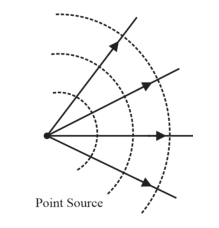(b) In case of a light emerging out of a convex lens when a point source is placed at focus, the shape of the wave front is parallel grid. This is shown in. the given figure.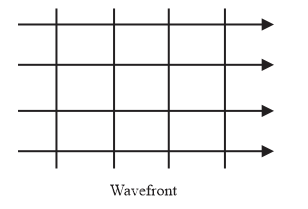(c) The portion of the wave-front of light from a distant star intercepted by the Earth is a plane.

Question 3. (a) The refractive index of glass is $1.5 .$ What is the speed of light in glass? (Speed of light in vacuum is $3.0 \times 10^{8} \mathrm{~m} \mathrm{~s}^{-1}$ )

(b) Is the speed of light in glass independent of the colour of light? If not, which of the two colours red and violet travels slower in a glass prism?

Solution. (a) Refractive index of glass, $\mu=1.5$

Speed of light, $\mathrm{c}=3 \times 10^{8} \mathrm{~m} / \mathrm{s}$

Speed of light in glass is,

$\mathrm{v}=\frac{\mathrm{c}}{\mu}$

$=\frac{3 \times 10^{8}}{1.5}=2 \times 10^{8} \mathrm{~m} / \mathrm{s}$

Hence, the speed of light in glass is $2 \times 10^{8} \mathrm{~m} / \mathrm{s}$.

(b) The speed of light in glass depends on the colour of light. The refractive index of a violet component of white light is higher than the refractive index of a red component of white light. Hence, the speed of violet light is lesser than the speed of red light in glass. Hence, violet light travels slower compared to red light in a glass prism.

Question 4. Young's double-slit experiment, the slits are separated by $0.28 \mathrm{~mm}$ and the screen is placed $1.4 \mathrm{~m}$ away. The distance between the central bright fringe and the fourth bright fringe is measured to be $1.2 \mathrm{~cm}$. Determine the wavelength of light used in the experiment.

Solution. Distance between the two slits, $\mathrm{d}=0.28 \mathrm{~mm}=0.28 \times 10^{-3} \mathrm{~m}$

Distance between the two slits and the screen, $D=1.4 \mathrm{~m}$

Distance between the central fringe and fourth $(\mathrm{n}=4)$ fringe,

$\mathrm{u}=1.2 \mathrm{~cm}=1.2 \times 10^{-2} \mathrm{~m}$

In case of constructive interference, we have the relation for the distance between the two fringes as:

$u=n \lambda \frac{D}{d}$

Where,

$n=$ order of fringes $=4$

$\lambda=$ wavelength of light used

$\therefore \lambda=\frac{u d}{n D}$

$=\frac{1.2 \times 10^{-2} \times 0.28 \times 10^{-3}}{4 \times 1.4}$

$=6 \times 10^{-7}$

$=600 \mathrm{~nm}$

Hence, the wavelength of the light is $600 \mathrm{~nm}$

Question 5. In Young's double-slit experiment using monochromatic light of wavelength $\lambda$, the intensity of light at a point on the screen where path difference is $\lambda$, is $\mathrm{K}$ units. What is the intensity of light at a point where path difference is $N 3$ ?

Solution. Let $I_{1}$ and $I_{2}$ be the intensity of the two light waves. The resultant intensities can be obtained as:

$I_{1}+I_{2}+2 \sqrt{I_{1} I_{2}} \cos \phi$

Where,

$\phi=$ Phase difference between the two waves

For monochromatic light waves,

$I_{1}=I_{2}$

$\therefore I^{\prime}=I_{1}+2 \sqrt{I_{1} I_{1}} \cos \phi$

$=2 I_{1}+2 I_{1} \cos \phi$

Phase difference $=\frac{2 \pi}{\lambda} \times$ Path difference

Since path difference $=\lambda$

Phase difference, $\phi=2 \pi$

$\therefore I^{\prime}=2 I_{1}+2 I_{1}=4 I_{1}$

Given

$I^{\prime}=K$

$\therefore I_{1}=\frac{K}{4} \ldots \ldots$ (1)

When path difference $=\frac{\lambda}{3}$,

Phase difference, $\phi=\frac{2 \pi}{3}$

Hence, resultant intensity, $\mathrm{I}_{\mathrm{R}}^{\prime}=\mathrm{I}_{1}+\mathrm{I}_{1}+2 \sqrt{\mathrm{I}_{1} \mathrm{I}_{1}} \cos \frac{2 \pi}{3}$

$=2 I_{1}+2 I_{1}\left(-\frac{1}{2}\right)=I_{1}$

Using equation (1), we can write:

$I_{R}=I_{1}=\frac{K}{4}$

Hence, the intensity of light at a point where the path difference is $\frac{\lambda}{3}$ is $\frac{K}{3}$ units.

Question 6. A beam of light consisting of two wavelengths, $650 \mathrm{~nm}$ and $520 \mathrm{~nm}$, is used to obtain interference fringes in a Young's double-slit experiment.

(a) Find the distance of the third bright fringe on the screen from the central maximum for wavelength $650 \mathrm{~nm}$.

(b) What is the least distance from the central maximum where the bright fringes due to both the wavelengths coincide?

Solution. The wavelength of the light beam, $\lambda_{1}=650 \mathrm{~nm}$

The wavelength of another light beam, $\lambda_{2}=520 \mathrm{~nm}$

The distance of the slits from the screen $=D$

Distance between the two slits $=d$

(a) Distance of the $n^{\text {th }}$ bright fringe on the sereen from the central maximum is, $x=n \lambda_{1}\left(\frac{D}{d}\right)$

For third bright fringe, $n=3$

$\therefore x=3 \times 650 \frac{D}{d}=1950\left(\frac{D}{d}\right) \mathrm{nm}$

(b) Let the $n^{\text {th }}$ bright fringe due to $\lambda_{2}$ and $(n-1)^{t h}$ bright fringe due to $\lambda_{1}$ coincide on the screen. So, we can equate the conditions for bright fringes as:

$n \lambda_{2}=(n-1) \lambda_{1}$

$520 n=650 n-650$

$650=130 n$

$\therefore n=5$

The least distance from the central maximum will be:

$x=n \lambda_{2} \frac{D}{d}$

$=5 \times 520 \frac{D}{d}=2600 \frac{D}{d}$

Question 7. In a double-slit experiment the angular width of a fringe is found to be $0.2^{\circ}$ on aa screen placed $1 \mathrm{~m}$ away. The wavelength of light used is $600 \mathrm{~nm}$. What will be the angular width of the fringe if the entire experimental apparatus is immersed in water? Take refractive index of water to be $4 / 3$.

Solution. The distance of the screen from the slits, $D=1 \mathrm{~m}$

The wavelength of light used, $\lambda_{1}=600 \mathrm{~nm}$

The angular width of the fringe in air $\theta_{1}=0.2^{\circ}$

The angular width of the fringe in water $\theta_{2}$

Refractive index of water, $\mu=\frac{4}{3}$

Refractive index of the medium is related to angular width as:

$\mu=\frac{\theta_{1}}{\theta_{2}}$

$\theta_{2}=\frac{3}{4} \theta_{1}$

$=\frac{3}{4} \times 0.2=0.15$

Therefore, the angular width of the fringe in water will reduce to $0.15^{\circ}$.

Question 8. What is the Brewster angle for air to glass transition? (Refractive index $=1.5$ )

Solution. Gievn

Refractive index of glass, $\mu=1.5$

Brewster angle $=\theta$

Brewster angle is related to the refractive index. Tangent angle of Brewster angle is equals of refractive angle.

$\tan \theta=\mu$

$\theta=\tan ^{-1}(1.5)=56.31^{\circ}$

Therefore, the Brewster angle for air to glass transition is $56.31^{\circ}$

Question 9. Light of wavelength 5000 A falls on a plane reflecting surface. What are the wavelength and frequency of the reflected light? For what angle of incidence is the reflected ray normal to the incident ray?

Solution. Wavelength of incident light, $\lambda=5000 A =5000 \times 10^{-10} \mathrm{~m}$

Speed of light, $c=3 \times 10^{8} \mathrm{~m}$

Frequency of Incident lights given by the relation,

$v=\frac{c}{\lambda}$

$=\frac{3 \times 10^{8}}{5000 \times 10^{-10}}=6 \times 10^{14} \mathrm{~Hz}$

The wavelength and frequency of incident light are equal to that of wavelength and frequency of reflected ray.

Hence, the wavelength of reflected light is $5000 \mathrm{~A}^{\circ}$ and its frequency is $6 \times 10^{14} \mathrm{~Hz}$

When reflected ray is perpendicular to Incident ray, the sum of the angle of Incidence,

$\angle i$ and angle of reflection, $\angle r$ is $90^{\circ}$

With the help of law of reflection, the angle of incidence is always equal to the angle of reflection. Hence, we can write the sum as:

$\angle i+\angle r=90$

$\angle i+\angle i=90$

$\angle i+\frac{90}{2}=45^{\circ}$

Therefore, the angle of incidence for the given condition is $45^{\circ}$.

Question 10. Estimate the distance for which ray optics is good approximation for an aperture of $4 \mathrm{~mm}$ and wavelength $400 \mathrm{~nm}$.

Solution. Fresnel's distance $\left(Z_{F}\right)$ is the distance for which the ray optics is a good approximation. If is given by the relation,

$Z_{F}=\frac{a^{2}}{\lambda}$

Where,

Aperture width, $a=4 \mathrm{~mm}=4 \times 10^{-3} \mathrm{~m}$

Wavelength of light, $\lambda=400 \mathrm{~nm}=400 \times 10^{-9} \mathrm{~m}$

$Z_{F}=\frac{\left(4 \times 10^{-3}\right)^{2}}{400 \times 10^{-9}}=40 \mathrm{~m}$

Therefore, the distance for which the optics is a good approximation is $40 \mathrm{~m}$

Question 11. The $6563 A \mathrm{H} \alpha$ line emitted by hydrogen in a star is found to be redshifted by 15 A. Estimate the speed with which the star is receding from the Earth.

Solution. Wavelength of $\mathrm{H}_{\alpha}$ line emitted by hydrogen,

$\lambda=6563 A$

$=6563 \times 10^{-10} \mathrm{~m}$

Star's red-shift, $\left(\lambda^{\prime}-\lambda\right)=15 A=15 \times 10^{-10} \mathrm{~m}$

speed of light, $\mathrm{c}=3 \times 10^{8} \mathrm{~m} / \mathrm{s}$

Let the velocity of the star receding away from the Earth be $\mathrm{v}$.

The red shift is related with velocity as:

$\lambda^{\prime}-\lambda=\frac{\mathrm{v}}{\mathrm{c}} \lambda$

$\mathrm{v}=\frac{\mathrm{c}}{\lambda} \times\left(\lambda^{\prime}-\lambda\right)$

$=\frac{3 \times 10^{8} \times 15 \times 10^{-10}}{6563 \times 10^{-10}}=6.87 \times 10^{5} \mathrm{~m} / \mathrm{s}$

Therefore, the speed of star while receding away from the Earth is $6.87 \times$ $10^{5} \mathrm{~m} / \mathrm{s}$

Question 12. Explain how Corpuscular theory predicts the speed of light in a medium, say, water, to be greater than the speed of light in vacuum. Is the prediction confirmed by experimental determination of the speed of light in water? If not, which alternative picture of light is consistent with experiment?

Solution. This prediction is not confirmed by experimental determination of the speed of light in water. Wave theory is consistent with the given experiment.

Newton's corpuscular theory of light states that when light corpuscles strike the Interface of two media from a rarer (air) to a denser (water) medium, the particles experience forces of attraction normal to the surface. Hence, the normal component of velocity increases while the component along the surface remains unchanged.

Hence, we can write the expression as:

$\operatorname{csin} \mathrm{i}=\operatorname{vsin} \mathrm{r} \ldots$.(i)

Where,

$\mathrm{I}=$ Angle of Incidence

$\mathrm{r}=$ Angle of regection

$\mathrm{c}=$ velocity of light in air

$\mathrm{v}=$ Velocity of light in water

Relative refractive Index of water with respect to air is given by the relation :

$\mu=\frac{V}{c}$

Hence, equation (i) will be

$\frac{\mathrm{v}}{\mathrm{c}}=\frac{\sin \mathrm{i}}{\sin \mathrm{r}}=\mu \ldots$ (ii)

But $\mu>1$

Hence, from equation (ii) we can say that $v>c$ which is not possible since this prediction is opposite to the experimental results of $\mathrm{c}>\mathrm{v}$.

The wave theory of light is consistent with the experimental results.

Question 13. You have learnt in the text how Huygens' principle leads to the laws of reflection and refraction. Use the same principle to deduce directly that a point object placed in front of a plane mirror produces a virtual image whose distance from the mirror is equal to the object distance from the mirror.

Solution. Let an object is placed at $\mathrm{O}$ in front of a plane mirror. $\mathrm{MO}^{\prime}$ at a distance $\mathrm{r}$ (as shown in the given figure).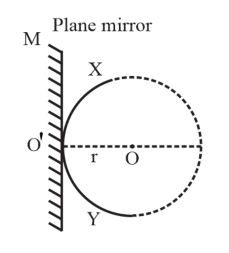circle is drawn with centre (0) such that it touches the plane mirror at point $0^{\prime}$. According to Huygens' Principle, wavefront of Incident light is XY. If the mirror is not present, then a similar wavefront $\mathrm{X}^{\prime} \mathrm{Y}^{\prime}$ (as $\mathrm{XY}$ ) would form behind $0^{\prime}$ at distance $\mathrm{r}$ (as Mown in the given figure).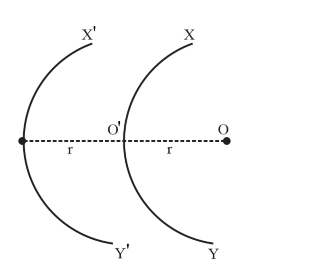we can consider $X^{\prime} Y^{\prime}$ a virtual reflected ray for the plane mirror. Hence, a point object which is placed in front of the plane mirror produces a virtual Image whose distance from the plane mirror is equal to the object distance (r).

Question 14. Let us list some of the factors, which could possibly influence the speed of wave propagation:

(i) nature of the source.

(ii) direction of propagation.

(iii) motion of the source and/or observer.

(iv) wavelength.

(v) intensity of the wave.

On which of these factors, if any, does

(a) the speed of light in vacuum,

(b) the speed of light in a medium (say, glass or water), depend?

Solution. (a) The speed of light in vacuum is $3 \times 10^{8} \mathrm{~m} / \mathrm{s}$ (approximately), which is a universal constant. The speed of light is not get affected by the motion of the source, the observer, or both. Hence, the speed of light in a vacuum is not affected by given factors.

(b) Among the given factors, the speed of light in a medium depends on the wavelength of light in that medium.

Question 15. For sound waves, the Doppler formula for frequency shift differs slightly between the two situations:

(i) source at rest; observer moving, and

(ii) source moving; observer at rest. The exact Doppler formulas for the case of light waves in vacuum are, however, strictly identical for these situations. Explain why this should be so. Would you expect the formulas to be strictly identical for the two situations in case of light travelling in a medium?

Solution. No

Sound waves can propagate only through a medium. The two given situations are not scientifically identical because Me motion of an observer relative to a medium is different in the two situations. Hence, the Doppler formulas for the two situations cannot be the same.

In case of light waves, sound can travel in a vacuum. In a vacuum, the above two cases are identical because the speed of light is independent of the motion of the observer a. the motion of the source. When light travels in a medium, the above two cases are not Identical because the speed of light depends on the wavelength of the medium.

Question 16. In double-slit experiment using light of wavelength $600 \mathrm{~nm}$, the angular width of a fringe formed on a distant screen is $0.1^{\circ} .$ What is the spacing between the two slits?

Solution: Wavelength width fringe, $\theta=0.1^{\circ}=0.1 \times \frac{\pi}{180}=\frac{3.14}{1800}$ rad

Angular width of a fringe is related to slit spacing (d) as:

$\theta=\frac{\lambda}{d}$

$d=\frac{\lambda}{d}$

$=\frac{600 \times 10^{-9}}{\frac{3.14}{1800}}=3.44 \times 10^{-4} \mathrm{~m}$

Therefore, the spacing between the slits is $3,44 \times 10^{-4} \mathrm{~m}$.

Question 17. Answer the following questions:

(a) In a single slit diffraction experiment, the width of the slit is made double the original width. How does this affect the size and intensity of the central diffraction band?

(b) In what way is diffraction from each slit related to the interference pattern in a double-slit experiment?

(c) When a tiny circular obstacle is placed in the path of light from a distant source, a bright spot is seen at the centre of the shadow of the obstacle. Explain why?

(d) Two students are separated by a $7 \mathrm{~m}$ partition wall in a room $10 \mathrm{~m}$ high. If both light and sound waves can bend around obstacles, how is it that the students are unable to see each other even though they can converse easily.

(e) Ray optics is based on the assumption that light travels in a straight line. Diffraction effects (observed when light propagates through small apertures/slits or around small obstacles) disprove this assumption. Yet the ray optics assumption is so commonly used in understanding location and several other properties of images in optical instruments. What is the justification?

Solution. (a) In a single slit diffraction experiment, if the width of the slit made double the original width, then the site of the central diffraction band gets reduced to half and the intensity of the central diffraction band gets Increased up to four times.

(b) The interference pattern in YDSE is modified by diffraction from each slot. The final pattern is the result of the interference of the diffracted wave from each slit.

(c) When a tiny circular obstacle is placed in the path of light from a distant source, a bright spot is seen at the centre of the shadow of the obstacle. This is because light waves are diffracted from the edge of the circular obstacle, which Interferes constructively at Me centre of the shadow. This constructive Interference produces a bright spot.

(d) Bending of waves by obstacles by a large angle is possible when the size of the obstacle Is comparable to the wavelength of the waves. On the one hand, the wavelength of the light waves is too small in comparison to the use of the obstacle. Thus, the diffraction angle will be very small. Hence, the students are unable to see each other. On the other hand, the size of the wall Is comparable to Me wavelength of Me sou. waves. Thus, Me bending of the waves takes place at a large angle. Hence, the students are able to hear each other.

(e) The justification is that in ordinary optical instruments, the size of the aperture involved Is much larger than the wavelength crone light used.

Question 18. Two towers on top of two hills are $40 \mathrm{~km}$ apart. The line joining them passes $50 \mathrm{~m}$ above a hill halfway between the towers. What is the longest wavelength of radio waves, which can be sent between the towers without appreciable diffraction effects?

Solution. Distance between the towers, $\mathrm{d}=40 \mathrm{~km}$

Height of the line pining the hills, $\mathrm{d}=50 \mathrm{~m}$.

Thus, the radial spread of the radio waves should not exceed $50 \mathrm{~km}$. Since the hill Is located halfway between the towers, Fresnel's distance can be obtained as:

$\mathrm{Z}_{\mathrm{p}}=20 \mathrm{~km}=2 \times 10^{4} \mathrm{~m}$

Aperture can be taken as:

$\mathrm{a}=\mathrm{d}=50 \mathrm{~m}$

Fresnel's distance is given by the relation,

$\therefore \mathrm{Z}_{\mathrm{p}}=\frac{\mathrm{a}^{2}}{\lambda}$

Where,

$\lambda=$ Wavelength of radio waves

$\therefore \lambda=\frac{\mathrm{a}^{2}}{\mathrm{Z}_{\mathrm{p}}}$

$=\frac{(50)^{2}}{2 \times 10^{4}}=1250 \times 10^{-4}$

$=0.1250 \mathrm{~m}=12.5 \mathrm{~cm}$

Therefore, the wavelength of the radio waves is $12.5 \mathrm{~cm}$

Question 19. A parallel beam of light of wavelength $500 \mathrm{~nm}$ falls on a narrow slit and the resulting diffraction pattern is observed on a screen $1 \mathrm{~m}$ away. It is observed that the first minimum is at a distance of $2.5 \mathrm{~mm}$ from the centre of the screen. Find the width of the slit.

Soluition. Wavelength of light beam, $\lambda=500 \mathrm{~nm}=500 \times 10^{-9} \mathrm{~m}$

Distance of the screen from the slit, $\mathrm{D}=1 \mathrm{~m}$

For first minima, $\mathrm{n}=1$

Distance of the first minimum from the centre of the screen can be denoted as:

$x=2.5 \mathrm{~nm}=2.5 \times 10^{-3} \mathrm{~m}$

It is related to the order of minima as:

$n \lambda=x \frac{d}{D}$

$\mathrm{d}=\frac{\mathrm{n} \lambda \mathrm{D}}{x}$

$=\frac{1 \times 500 \times 10^{-9} \times 1}{2.5 \times 10^{-3}}=2 \times 10^{-4} \mathrm{~m}=0.2 \mathrm{~mm}$

Therefore, the width of the slits is $0.2 \mathrm{~mm}$.

Question 20. Answer the following questions:

(a) When a low flying aircraft passes overhead, we sometimes notice a slight shaking of the picture on our TV screen. Suggest a possible explanation.

(b) As you have learnt in the text, the principle of linear superposition of wave displacement is basic to understanding intensity distributions in diffraction and interference patterns. What is the justification of this principle?

Solution: (a) Weak radar signals sent by a low flying aircraft can Interfere with the TV signals received by the antenna. As a result, the TV signals may get distorted. Hence, when a low flying aircraft passes overhead, we sometimes notice a slight shaking of the picture on our TV screen.

(b) The principle of linear superposition of wave displacement is essential to our understanding of intensity distributions and interference patterns. This is because superposition follows from the linear character of a differential equation that governs wave motion. If $y_{1}$ and $y_{2}$ are the solutions of the second order wave equation, then an linear combination of $y_{1}$ and $y_{2}$ will also be the solution of the wave equation.

Question 21. In deriving the single slit diffraction pattern, it was stated that the intensity is zero at angles of $n \frac{\lambda}{a}$. Justify this by suitably dividing the slit to bring out the cancellation.

Solution: Consider that a single slit of width $\mathrm{d}$ is divided into $\mathrm{n}$ smaller slits.

$\therefore$ Width of each slit, $d^{\prime}=\frac{d}{n}$

Angle of diffraction is giving by the relation,

$\theta=\frac{\frac{d}{d} \lambda}{d}=\frac{\lambda}{d}$

Each of this infinitesimally small slit sends zero intensity in direction $\theta$. Hence, the combination of these slits will give zero intensity.